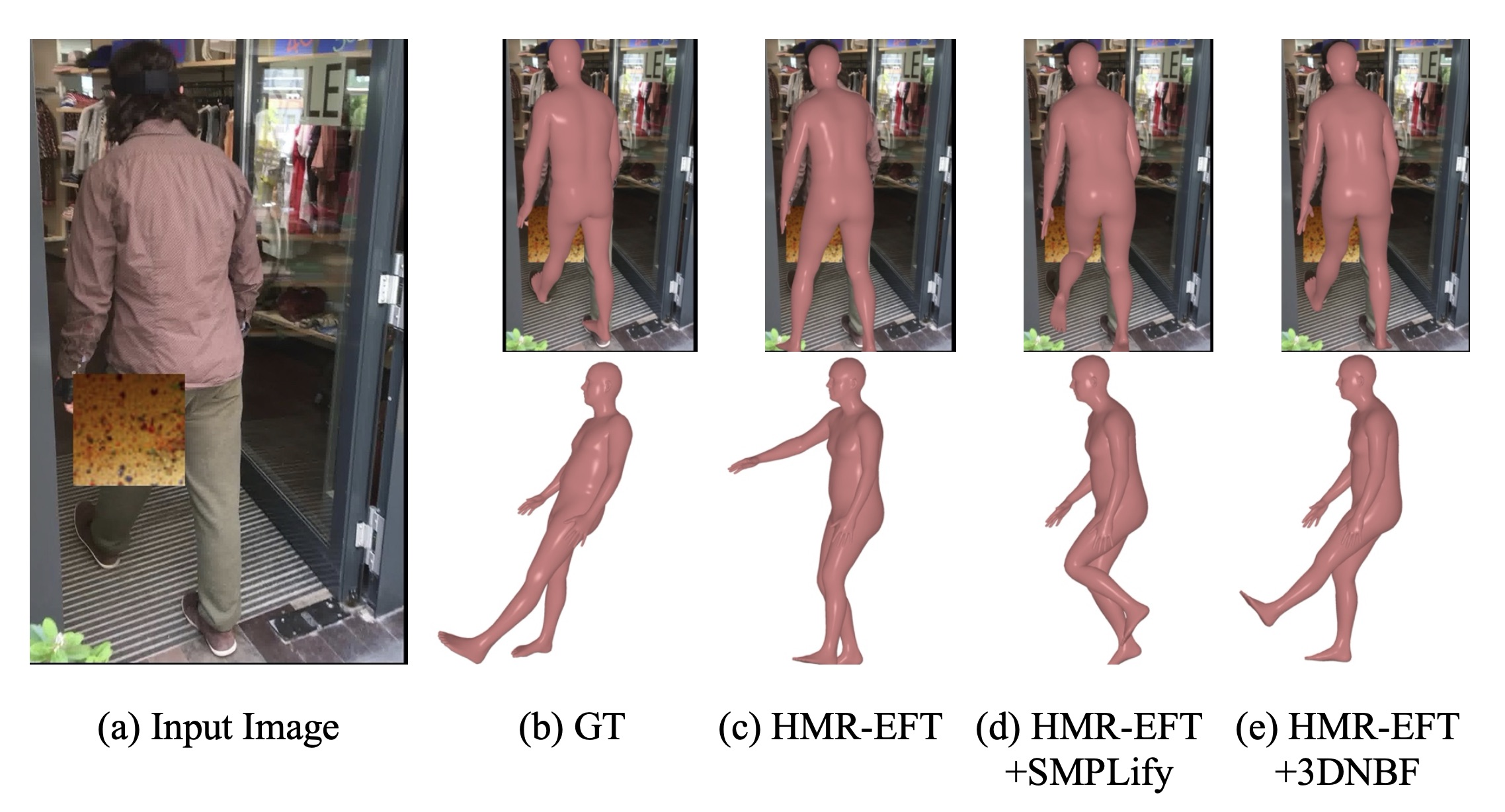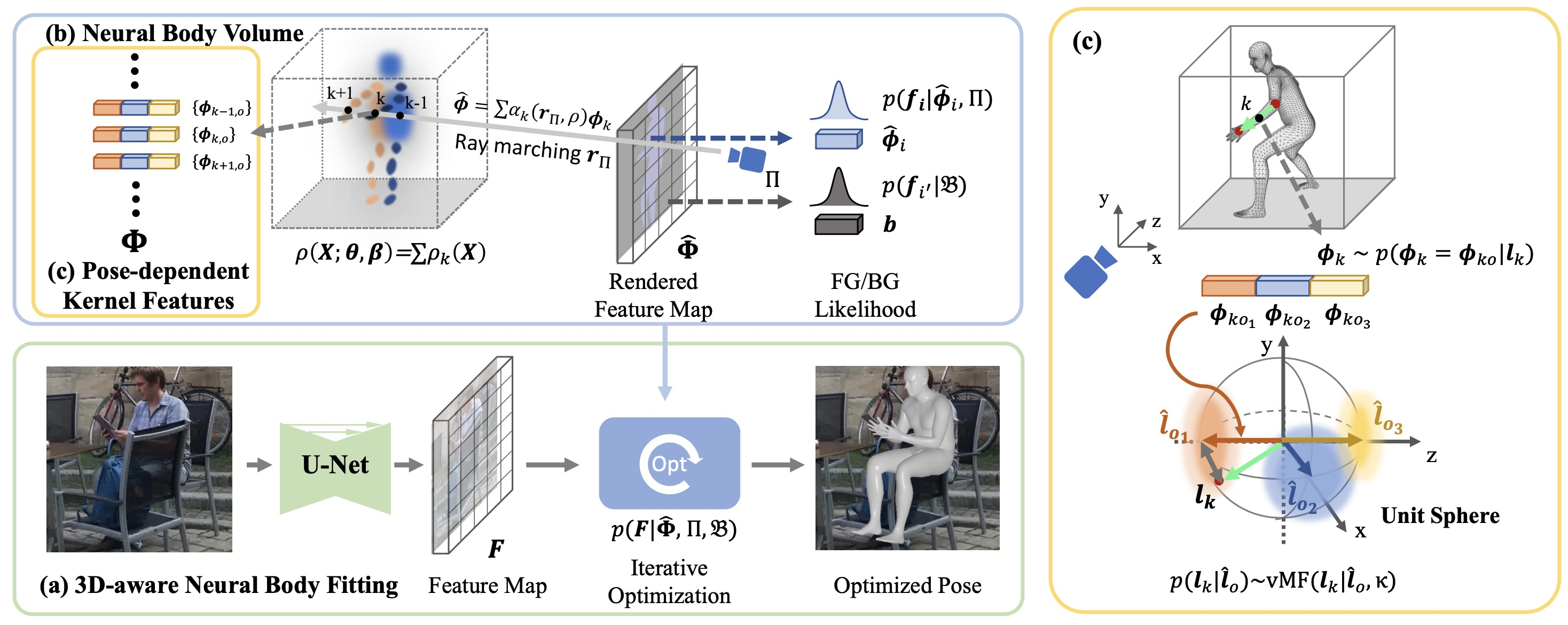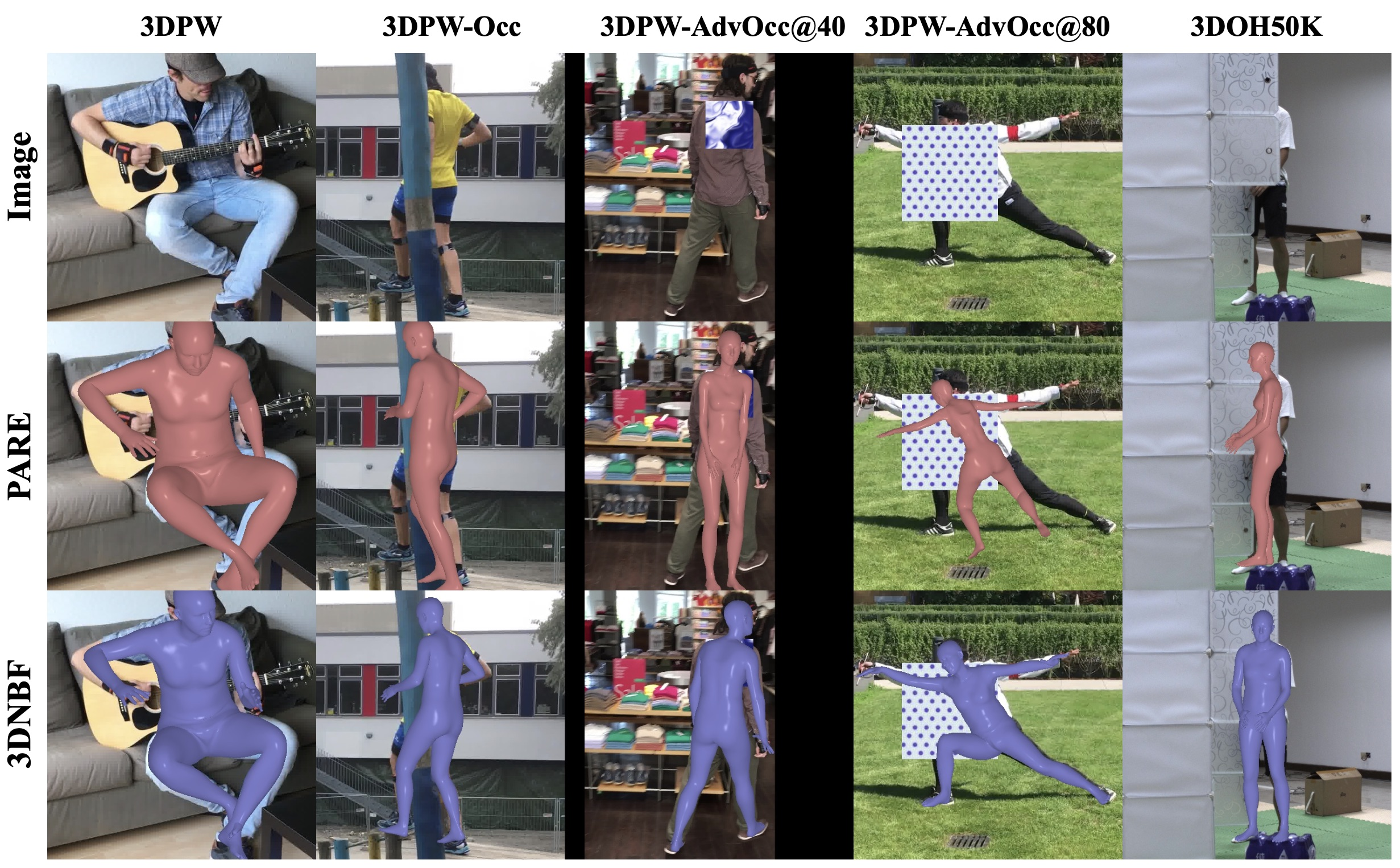# 3D-Aware Neural Body Fitting for Occlusion Robust 3D Human Pose Estimation

ICCV 2023
1Johns Hopkins University, 2Beihang University,
3Max Planck Institute for Informatics, 4University of Freiburg## Abstract

Regression-based methods for 3D human pose estimation directly predict the 3D pose parameters from a 2D image using deep networks. While achieving state-of-the-art performance on standard benchmarks, their performance degrades under occlusion. In contrast, optimization-based methods fit a parametric body model to 2D features in an iterative manner. The localized reconstruction loss can potentially make them robust to occlusion, but they suffer from the 2D-3D ambiguity.

Motivated by the recent success of generative models in rigid object pose estimation, we propose 3D-aware Neural Body Fitting (3DNBF) - an approximate analysis-by-synthesis approach to 3D human pose estimation with SOTA performance and occlusion robustness. In particular, we propose a generative model of deep features based on a volumetric human representation with Gaussian ellipsoidal kernels emitting 3D pose-dependent feature vectors. The neural features are trained with contrastive learning to become 3D-aware and hence to overcome the 2D-3D ambiguity.

Experiments show that 3DNBF outperforms other approaches on both occluded and standard benchmarks.

## Method(a) We perform feature-level analysis-by-synthesis for 3D human pose estimation by fitting a 3D-aware generative model of deep feature (NBV) to the feature map $$\mathbf{F}$$ extracted by a U-Net. (b) NBV is defined as a volume representation of human body $$\rho$$, driven by pose and shape parameters $$\{\mathbf{\theta}, \mathbf{\beta}\}$$, which consists of a set of Gaussian kernels each emitting a pose-dependent feature $$\mathbf{\phi}$$. Volume rendering is used to render NBV to a feature map $$\mathbf{\hat{\Phi}}$$. The foreground feature likelihood is defined as a Gaussian distribution centered at the rendered feature vector while the background feature likelihood is modeled by a background model. Pose estimation is done by optimizing the negative log-likelihood (NLL) loss of $$\mathbf{F}$$ w.r.t. $$\{\mathbf{\theta}, \mathbf{\beta}\}$$ and camera $$\Pi$$. (c) the distribution of the kernel feature is conditioned on the orientation of the limb that the kernel belongs to.

## Qualitative ResultsQualitative results on non-occluded (3DPW) and occluded (3DPW-OCC, 3DPW-AdvOcc, 3DOH50K) benchmarks.

## BibTeX

@article{zhang2023nbf,
author    = {Zhang, Yi and Ji, Pengliang and Wang, Angtian and Mei, Jieru and Kortylewski, Adam and Yuille, Alan L},
title     = {3D-Aware Neural Body Fitting for Occlusion Robust 3D Human Pose Estimation},
journal   = {ICCV},
year      = {2023},
}